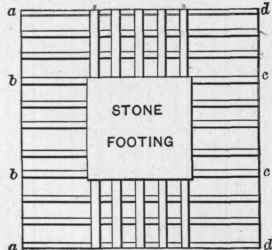## 56. B. Beams Under Piers (Fig. 16)

In this case the size of the lower beams are determined in the same way as in Example I., the length of p being taken from the end of the beam to the centre of the outer beam in upper tier.

For the upper beams the load borne by each beam should be computed and the coefficient of strength determined by the formula

C=4X WX p.........(2)

W being in this case the total distributed load on either end of the beam in pounds, and p the distance from end of beam to edge of iron plate above.

Example II. - The basement columns of a ten-story building are required to sustain a permanent load of 400,000 pounds. What should be the size of the beams in the footings, the supporting power of the soil being but 2 tons?

Answer. - Dividing the load by the bearing power of the soil we have 100 square feet, or 10X10 feet, for the area of the footing. We will arrange the beams as shown in Fig. 16, using a cast iron bearing plate 3 feet square under the column. The distance between the centres of outer beams in upper tier we will make 32 inches, thus making the value of p for the lower beams = 10' - 2' 8" / 2 or 3 2/3 feet; s we will make 12 inches, or 1.PLAN.SECTION.

Fig. 16.

Looking down column headed 2 (Table IV.) we find the nearest projection above 3 2/3 is 4, which is opposite the 9-inch, 27-pound and also the 10-inch, 25.5-pound beams. The latter being the lighter and also the stiffer, we will use for the lower tier.

For the upper tier we see that the five beams must support an area equal to a, b, c, d, which in this case equals 3½ X 10 feet, or 35 square feet. As the pressure on each foot is 2 tons, we will have a total pressure of 70 tons on the ends of the five beams, or 14 tons or 28,000 pounds on each beam. Then by formula 2 we find the coefficient of strength must =4X28,000X3½=392,000 pounds.

From the table of the Carnegie Steel Company's beams we find that the coefficient for a 12-inch, 32-pound beam is 395,200 pounds; therefore, we will use three 12-inch, 32-pound beams and two

40-pound beams in the upper tier.

57. The deepest beam for the weight should always be used, and unless the beams in the upper tier have considerable excess of strength, the two outer beams should be heavy beams.

When the footings carry iron or steel columns in the basement, as is generally the case, a cast iron or steel base plate should be used, as shown in Figs. 17 and 18. This plate should be bedded in Portland cement directly above the beams, as described in Section 50.

Two and even four columns are often supported on one footing, as shown in Figs. 17 and 18. In such cases the computation becomes more elaborate, and an engineer should be called into consultation unless the architect is himself sufficiently familiar with such calculations.

Fig. 19 shows an arrangement in which a built-up base plate or girder is used in place of the upper tier of beams. The author believes this arrangement much better than that shown in Figs. 16 to 18.Fig. 17.

In placing the beams, it is essential that they be arranged symmetrically under the base plate, otherwise they will sink more at one side than at the other. When several unequally loaded columns rest on the same footing, the equal distribution of the weight on the soil becomes a difficult problem.